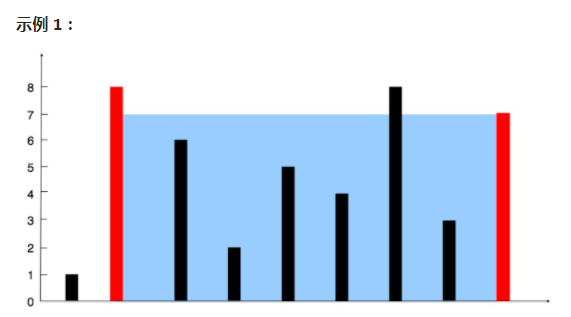﻿• 价格透明
• 信息保密
• 进度掌控
• 售后无忧# 20210407力扣11题：11. 盛最多水的容器JAVA

## 1.题目描述``````输入：[1,8,6,2,5,4,8,3,7]

``````

## 2.自己想法

``````class Solution {
public int maxArea(int[] height) {
int area = 0;//面积
int temp = 0;//临时面积
for(int i = 0;i<height.length;i++)
{
for(int j = i+1;j<height.length;j++)
{
if(height[i]<height[j])
{
temp = height[i]*(j-i);
}
else
{
temp = height[j] * (j-i);
}
if(temp > area) area = temp;
}
}
return area;
}
}
``````

## 3.别人思路

``````class Solution {
public int maxArea(int[] height) {
int i = 0;
int j = height.length-1;
int area = 0;
while(i<j){
/*area = height[i]<height[j]?
Math.max(area,(j-i)*height[i++])
:Math.max(area,(j-i)*height[j--]);*/
if(height[i]<height[j]){
area = Math.max(area,(j-i)*height[i]);
i++;
}
else{
area = Math.max(area,(j-i)*height[j]);
j--;
}
}
return area;

}
}
``````

## 4.总结

``````public int maxArea(int[] a) {
int max = 0;
for(int i = 0, j = a.length - 1; i < j ; ){
int minHeight = a[i] < a[j] ? a[i ++] : a[j --];
max = Math.max(max, (j - i + 1) * minHeight);
}
return max;
}
``````

``````or(int i = 0, j = a.length - 1; i < j ;i++,j-- ){
int minHeight = a[i] < a[j] ? a[i ] : a[j ];
max = Math.max(max, (j - i + 1) * minHeight);
``````

``````	留下疑问：1.为什么for循环那个 i++ ，j-- 有问题
``````### 低价透明### 金牌服务### 信息保密